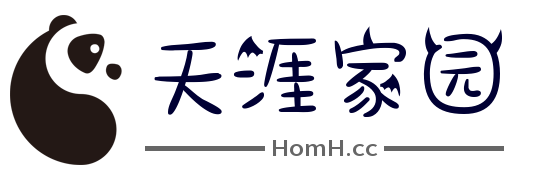# 天涯家园HomeH```<?php
\$x=5;
\$y=6;
\$z=\$x+\$y;
echo \$z;
?>```

```x=5
y=6
z=x+y```

## PHP 变量

### PHP 变量规则：

• 变量以 \$ 符号开头，其后是变量的名称

• 变量名称必须以字母或下划线开头

• 变量名称不能以数字开头

• 变量名称只能包含字母数字字符和下划线（A-z、0-9 以及 _）

• 变量名称对大小写敏感（\$y 与 \$Y 是两个不同的变量）

## 创建 PHP 变量

PHP 没有创建变量的命令。

### 实例

```<?php
\$txt="Hello world!";
\$x=5;
\$y=10.5;
?>```

## PHP 是一门类型松散的语言

PHP 根据它的值，自动把变量转换为正确的数据类型。

PHP 有三种不同的变量作用域：

• local（局部）

• global（全局）

• static（静态）

## Local 和 Global 作用域

### 实例

```<?php
\$x=5; // 全局作用域function myTest() {
\$y=10; // 局部作用域
echo "<p>测试函数内部的变量：</p>";
echo "变量 x 是：\$x";
echo "<br>";
echo "变量 y 是：\$y";
}

myTest();

echo "<p>测试函数之外的变量：</p>";
echo "变量 x 是：\$x";
echo "<br>";
echo "变量 y 是：\$y";
?>```

## PHP global 关键词

global 关键词用于在函数内访问全局变量。

### 实例

```<?php
\$x=5;
\$y=10;

function myTest() {  global \$x,\$y;
\$y=\$x+\$y;
}

myTest();
echo \$y; // 输出 15?>```

PHP 同时在名为 \$GLOBALS[index] 的数组中存储了所有的全局变量。下标存有变量名。这个数组在函数内也可以访问，并能够用于直接更新全局变量。

### 实例

```<?php
\$x=5;
\$y=10;

function myTest() {
\$GLOBALS['y']=\$GLOBALS['x']+\$GLOBALS['y'];
}

myTest();
echo \$y; // 输出 15?>```

## PHP static 关键词

### 实例

```<?php

function myTest() {  static \$x=0;
echo \$x;
\$x++;
}

myTest();
myTest();
myTest();

?>```

查看权限

### 网站收藏

«    2023年2月    »
12345
6789101112
13141516171819
20212223242526
2728